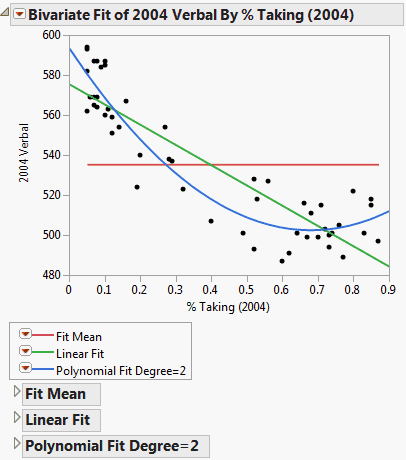Basic Analysis > Bivariate Analysis > Fit Line and Fit Polynomial
Publication date: 08/13/2020

# Fit Line and Fit Polynomial

Using the Fit Line option, you can add straight line fits to your scatterplot using least squares regression. Using the Fit Polynomial option, you can fit polynomial curves of a certain degree using least squares regression.

Figure 5.8 Example of Fit Line and Fit PolynomialFigure 5.8 shows an example that compares a linear fit to the mean line and to a degree 2 polynomial fit.

Note the following information:

The Fit Line output is equivalent to a polynomial fit of degree 1.

The Fit Mean output is equivalent to a polynomial fit of degree 0.